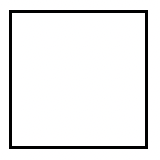### Home > AC > Chapter 8 > Lesson 8.1.3 > Problem8-28

8-28.Remember that a square is a rectangle with four equal sides.

1. If a square has an area of $81$ square units, how long is each side?

Take the square root of the area to find the length of each side.

2. Find the length of the side of a square with area $225$ square units.

Use the same strategy as part (a).

3. Find the length of the side of a square with area $10$ square units.

Use the same strategy as part (a).

$\sqrt {10} \text{ units}$

4. Find the area of a square with side $11$ units.

$\text{Area} = \left(\text{length}\right) \left(\text{width}\right)$